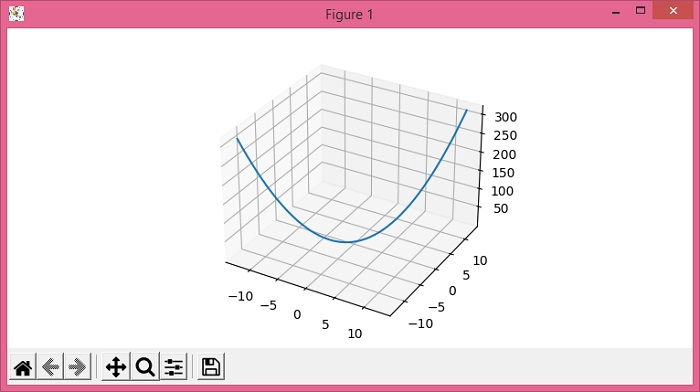# How to plot a 3D continuous line in Matplotlib?

To plot a 3D continuous line in Matplotlib, we can take the following steps −

• Set the figure size and adjust the padding between and around the subplots.

• Create x and y data points using numpy.

• Create z data points using x and y data points.

• Create a new figure or activate an existing figure using figure() method.

• Add an axes as a subplot arrangement with 3D projection.

• Plot x, y and z data points using plot() method.

• To display the figure, use show() method.

## Example

import numpy as np
from matplotlib import pyplot as plt
plt.rcParams["figure.figsize"] = [7.50, 3.50]
plt.rcParams["figure.autolayout"] = True
x = np.linspace(-4 * np.pi, 4 * np.pi, 50)
y = np.linspace(-4 * np.pi, 4 * np.pi, 50)
z = x ** 2 + y ** 2
fig = plt.figure()
plt.show()